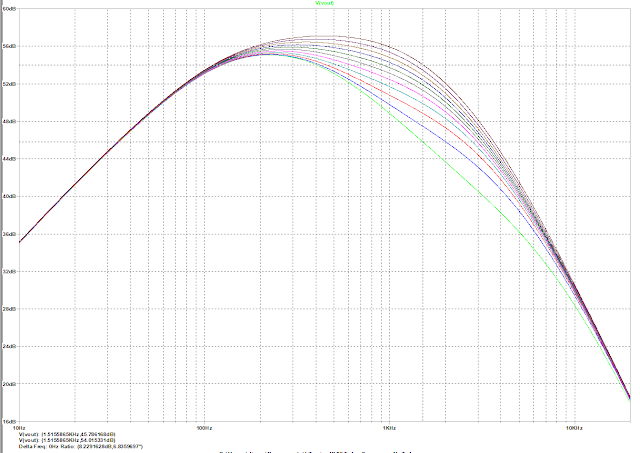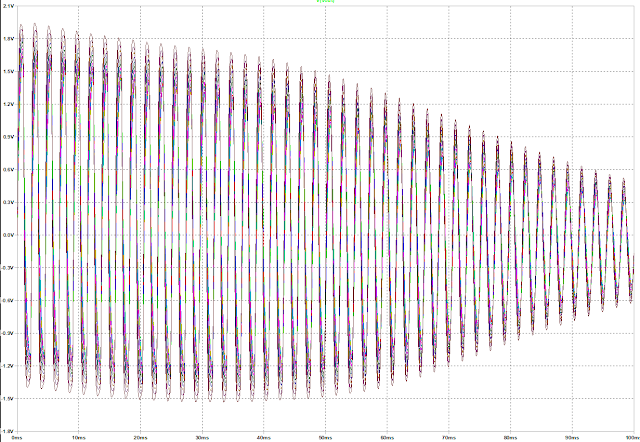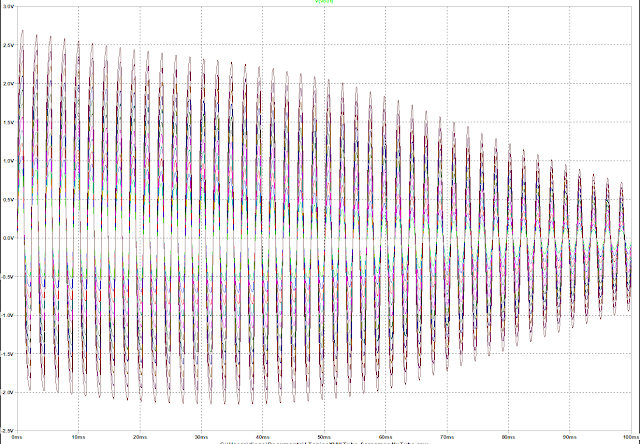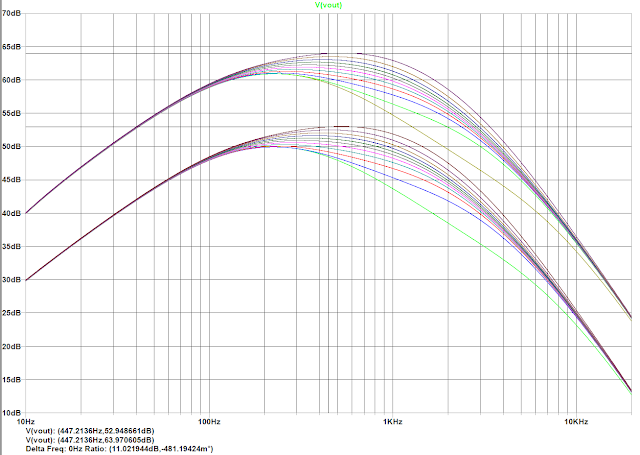## NuTube Overdrive Schematics

NuTube Overdrive is basically a Tube Screamer TS808 classic circuit (with a couple of mods I will explain later) where two NuTube triodes amplifier stages have been added between the preamp and the tone circuit, the original Tube Screamer output of the preamp is mixed with the output of the two triode amp sections by means of a 100K linear potentiometer, the buffered output of the mix is connected to the original tone circuit.

The following figure shows the LTSpice schematics of the NuTube Screamer pedal:NuTube Screamer LTSpice schematics

## NuTube Screamer Mods

The following mods have been made to the original schematics:
1. Bass boost mod: 220nF capacitor added in parallel to 47nF on the high pass filter connected to the preamp section inverting input. This mod can be remove by just removing a series 0R resistor
2. Tone mod: 20K tone potentiometer has been replaced by 5K linear potentiometer providing a more gradual response of the tone potentiometer.
3. Asymmetric clipping: The original pedal had symmetric clipping, here an additional diode has been added in series to the positive output cycle.
4. Output buffer: Resistor biasing has been slightly changed using 1000K resistor bridge from +9VDC instead of a 510K single resistor to +4.5VDC. It is basically the same.
Some components have been changed due to availability, performance or space gain:
1. Original diodes were silicon MA150, they have been changed by dual BAV199 silicon diodes for space gain, and easier asymmetrical circuit construction.
2. Original opamp JRC4558 has been replaced by LME49723 which has better performance in terms of harmonic distortion, noise, bandwidth, offset voltage and slew rate. See comparison here
3. Original transistors 2SC1815 used for buffers  have been replaced by MMBT5089 in a small SOT23 package

## Frequency response

The following figure shows the gain response from 0 to 10 with tone at 5 and mix at 10, maximum gain is 56 dB and minimum gain is 36 dB:NuTube Screamer Gain = 0 to 10, Tone = 5, Mix = 10
The following figure shows tone response from 0 to 10 with gain at 10 and mix at 10:NuTube Screamer Tone = 0 to 10, Gain = 10, Mix = 10
The following figure shows Mix response from 0 to 10 with gain at 10 and tone at 5, maximum gain is 56 dB and minimum gain is 38 dB:NuTube Screamer Mix = 0 to 10, Gain 10, Tone = 5
The total gain of NuTube triode amps section is 17.7dB, 8.2 dB for the first section, almost flat from 10 Hz to 20KHz (82mdB loss at 20 kHz) and 9.5dB for the second section (with 141dB loss at 20kHz)

## Time response

Time response shows the pedal output signal with different settings with a 60 mVp-p 30s-1 decaying 440Hz sinewave input signal. Clipping is always on.

The following figure shows Gain response from 0 to 10 with Tone at 5 and Mix at 5:NuTube Screamer time response with Gain = 0 to 10, Mix = 10, Tone = 5
The following figure shows Tone response from 0 to 10 with Gain at 10 and Mix at 5:NuTube Screamer time response with Tone = 0 to 10, Mix = 5, Gain = 10
The following figure shows Mix response from 0 to 10 with Gain at 10 and Tone at 5:NuTube Screamer time response with Mix = 0 to 10, Tone = 5, Gain = 10

### Frequency response at +9V and +18V

The same circuit could be powered at +18V, to increase around 10dB the gain, here are the simulations at +9V and +18VNuTube Screamer Freq response at +9V and +18V with Tone = 0 to 10, Gain = 10, Mix = 10

#### 1 comment:

1.The article is much informative which i was searching for .Nice intro good explanation thanks for sharing.
Enrgtech